Introduction to Digital Circuits : 3 of 3

In this session – Part 3 of Digital Circuits, we will look at The Flip-Flop Circuit & Sequential Logic circuit

## What is a Sequential Logic Circuit?

In our last session we saw how combinational circuits produce a single output from a combination of input signals. A combinational circuit does not have memory as its output is determined only by the present input, and not by any previous input. The output cannot reflect previous input level conditions.

In contrast, sequential circuits—the subject of this session—do have memory. The output of a sequential circuit is determined both by the present input and previous input.

What specifically does a sequential circuit need in order to reflect past input into its present output? Clearly, it needs a memory element. Such a memory element is called a flip-flop.

There are four basic types of flip-flips, as determined by structure and operation: RS, JK, D, and T. In this session we will look at RS and D types.

## Why Do We Call Them " Flip-Flops" ?

Because they flip between two stable states, in the manner of a seesaw that comes to rest on one end or the other. So let’s use this analogy to help explain the operation of an RS flip-flop.

Consider the seesaw shown in Figure 2. It’s a bit rusty, and will remain at rest on either end when there’s no one on it. Now note the following: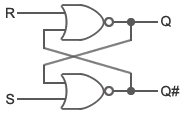Figure 1: An RS Flip-Flop Circuit

• The left end of the seesaw represents output Q; the right end, output Q#.
• The people—R and S—represent the inputs. The input goes to logical H when a person gets on the seesaw, and to L when a person gets off. (The analogy is not perfect: We do not allow R and S to be on the seesaw at the same time!)Figure 2: Initial State of Seesaw (Q = L, Q# = H, R = L, S = L)

Figure 3 shows what happens when S gets on: input S goes to H, Q goes to H, and Q# goes to LFigure 3: State of Seesaw when S gets on (Q = H, Q# = L, R = L, S = H)

The seesaw has stopped moving, even if S gets off (S = L). Q# stays L. (Fig. 4.)Figure 4: State of Seesaw After S Gets On and Then Off (Q = H, Q# = L, R = L, S = L)

Suppose that R got on instead of S. In that case Q would be L (Q# would be H); and this state would remain after R gets off. We can see how the movement of the seesaw " remembers" which person was previously on board.

The truth table in Figure 5 shows how the RS flip-flop operates. In the table, Q0 and Q0# are the outputs in effect before the input change.

The RS flip-flop is the simplest of the four flip-flop types. It’s often used, for example, to prevent malfunctions of mechanical switches.

Operation Set Reset Hold S R Q Q# H L H L L H L H L L Q0 Q0# H H L L

Figure 5: Truth Table of the RS Flip-Flop

## Clocked D Flip-Flop

The D flip-flop captures the value of the D input at the time of the rising edge (L→H) and/or falling edge (H→L) of the incoming clock pulse (CK), and outputs this value from the Q output.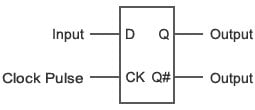Figure 6: D Flip-Flop

Input Output
CK D Q Q#
L L H
H H L
Not ↑ x Q0 Q0#

Q0：Output before input changes
x：Either H or L
↑：Transition from L to H
Figure 7: Truth Table of the D Flip-Flop

Let’s reuse the seesaw analogy to explain the D flip-flop. Figure 8 shows an initial state. Input D goes to H when Mr. D gets on the seesaw; it goes to L when Mr. D gets off. A weight that is lighter than Mr. D rests on the other side of the seesaw. And unlike a real seesaw, the seesaw we are imagining can change its state only at the moment when it is receiving the rising edge of the clock pulse.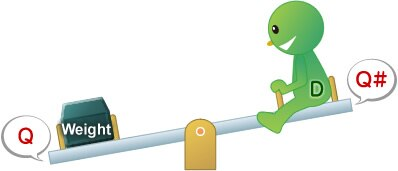Figure 8: Initial State of D Flip-Flop (CK = L, D = H, Q = L, Q# = H)

Figure 8 looks a bit strange: Although Mr. D is heavier than the weight, he is still on the up side, with the weight on the downside. The reason, of course, is that CK is still at L. When CK goes H (at the rising edge of the signal), Mr. D’s side will come down—as shown in Figure 9.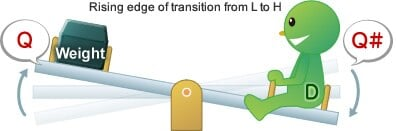Figure 9: State of D Flip-Flop After CK goes H (D = H, Q = H, Q# = L)

Now that Mr. D’s side is down, it must stay down at least until the next time that the CK signal changes from L to H. In other words, the seesaw " remembers" its state at least until CK completes both its current H cycle and subsequent L cycle, regardless of how many times Mr. D jumps off and gets back on in the meantime.

The D flip-flop, in other words, is a clock-synchronized sequential logic circuit that remembers the state in effect during the instant that the CK signal last changed from L to H.

D flip-flops are a basic building block of sequential circuitry, and have a wide range of uses. They can be configured together in multiple stages to implement shift registers and clock division circuits. They are also found in internal CPU registers.

### Flip-Flops and SRAM

A flip-flop can store 1 bit of information: either it’s at H or it’s at L. Accordingly, SRAM can be implemented by arraying together a large number of flip-flops and adding the necessary select capability. Because SRAM works much faster than DRAM and flash memory, it is commonly used to build CPU caches and registers.

In practice, CPU memory and registers do not use logic gates—such as those built with RS flip-flops—because the circuitry gets too big. This memory is typically implemented using four or six FETs per bit. (Fig. A).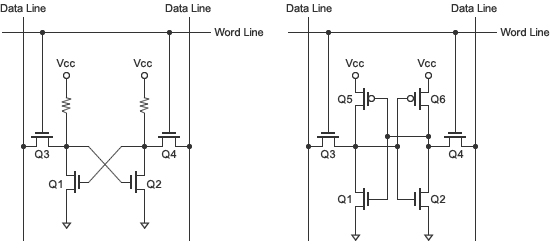Figure A: Basic SRAM Circuit

## The Need for Clocked Circuits

Over the last two sessions, we have explained the basics of logic circuits. In our previous session, we looked at combinational circuits, and this time we’ve looked at sequential circuits. To conclude this session, we’d like to point out that there are many considerations that must be taken into account when designing logic circuitry. One of the more important issues relates to the use of clocked circuits.

In particular, it sometimes happens that a combinational circuit will output a very short unwanted signal as a result of a slight delay in signal transmission. This unwanted output, which is appropriately called a glitch, can produce errors in the logic circuitry. The problem can be prevented by building in clocked circuitry, as illustrated below.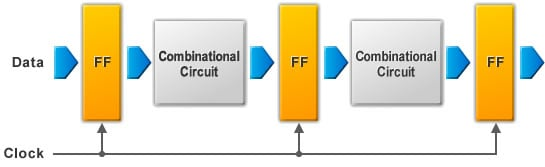Figure 10: Using Clocked Circuits to Prevent Glitch

Figure 10 shows how clocked circuitry is included. As you can see, the combinational circuits are sandwiched between flip-flops (FF).

A glitch survives only during the short interval before the combinational circuit’s output stabilizes. To prevent a glitch: Wait for the output to stabilize, and then change the clock, causing the flip-flop to capture the intended output value.

With this session, we conclude our introduction to digital circuitry. We began the series by defining what we mean by " digital," and then proceeded quickly through a discussion of basic logic circuits, digital ICs, combinational circuits, and—finally—sequential circuits.

Of course, we’ve only touched the surface, and there’s still a tremendous amount to be learned about the practice of circuit design. We encourage you to make time to continue studying this area on your own. And we look forward to seeing you at the next session, where we will begin with an introduction to microcontrollers.

In our next session we begin our introduction to microcontrollers. We look forward to seeing you there.

## Module List

1. Elemental Digital Circuits
2. Digital ICs/Combinational Logic
3. Sequential Logic